# GPvecchia tutorial

#### 2020-04-21

In this vignette, we will demonstrate the main capabilities of the GPvecchia package. These include estimating parameters and making spatial predictions. We also show how to use the package for processing non-linear, non-Gaussian data by combining the Vecchia with a Laplace approximation.

We start by importing the GPvecchia library.

library(GPvecchia)
library(Matrix)
library(fields)
#> Spam version 2.5-1 (2019-12-12) is loaded.
#> Type 'help( Spam)' or 'demo( spam)' for a short introduction
#> and overview of this package.
#> Help for individual functions is also obtained by adding the
#> suffix '.spam' to the function name, e.g. 'help( chol.spam)'.
#>
#> Attaching package: 'spam'
#> The following object is masked from 'package:Matrix':
#>
#>     det
#> The following objects are masked from 'package:base':
#>
#>     backsolve, forwardsolve
#> See https://github.com/NCAR/Fields for
#>  an extensive vignette, other supplements and source code

### Simulating data for illustration

To illustrate our method, we simulate a small dataset. First, consider a unit square and randomly select observation locations. (Set spatial.dim=1 to consider data on the one-dimensional unit interval.)

set.seed(1988)
spatial.dim=2
n=50
if(spatial.dim==1){
locs=matrix(runif(n),ncol=1)
} else {
locs <- cbind(runif(n),runif(n))
}

Next, we define the covariance function of the field as well as the scale of the measurement error

beta=2
sig2=1; range=.1; smooth=1.5
covparms =c(sig2,range,smooth)
covfun <- function(locs) sig2*MaternFun(fields::rdist(locs),covparms)
nuggets=rep(.1,n)

We are now ready to simulate the field and visualize it as a sanity check.

Om0 <- covfun(locs)+diag(nuggets)
z=as.numeric(t(chol(Om0))%*%rnorm(n))
data=z+beta

# plot simulated data
if(spatial.dim==1) {
plot(locs,data)
} else {
fields::quilt.plot(locs,data, nx=n, ny=n)
}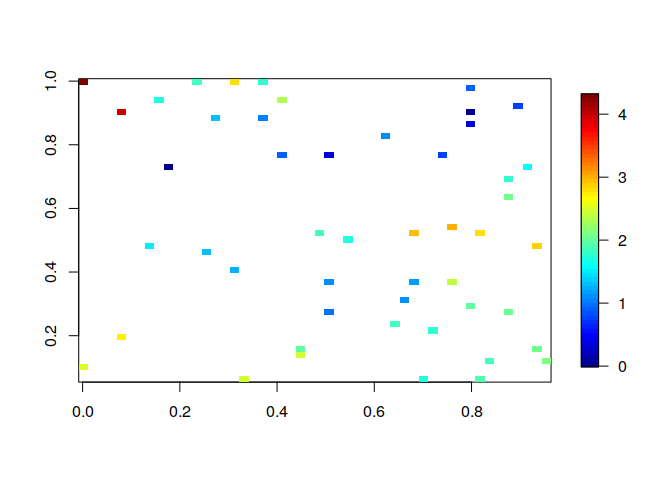We also create a grid of $$n.p$$ locations at which we would like to make predictions.

n.p=100
if(spatial.dim==1){  #  1-D case
locs.pred=matrix(seq(0,1,length=n.p),ncol=1)
} else {   # 2-D case
grid.oneside=seq(0,1,length=round(sqrt(n.p)))
locs.pred=as.matrix(expand.grid(grid.oneside,grid.oneside)) # grid of pred.locs
}
n.p=nrow(locs.pred)

### Basic functions for parameter estimation and prediction

Let us now estimate the mean and covariance parameters using the default settings, which assume a spatially constant mean or trend, and a Matern covariance structure. Note that the following code might take a minute or so to run.

vecchia.est=vecchia_estimate(data,locs,,output.level=0)

Based on these parameter estimates, we can then make predictions at the grid of locations we had specified above.

preds=vecchia_pred(vecchia.est,locs.pred)

Finally, we compare the approximate predictions with the best possible ones (i.e. those obtained using analytic expressions for conditional mean in the Gaussian distribution).

##  exact prediction
mu.exact=as.numeric(covfun(rbind(locs,locs.pred))[,1:n]%*%solve(Om0,data-beta))+beta
cov.exact=covfun(rbind(locs,locs.pred))-
covfun(rbind(locs,locs.pred))[,1:n]%*%solve(Om0,t(covfun(rbind(locs,locs.pred))[,1:n]))
var.exact=diag(cov.exact)
cov.exact.pred=cov.exact[n+(1:n.p),n+(1:n.p)]

### plot Vecchia and exact predictions
if(spatial.dim==1) {
plot(locs,z)
lines(locs.pred,preds$mean.pred,col='blue') lines(locs.pred,preds$mean.pred-1.96*sqrt(preds$var.pred),col='blue',lty=2) lines(locs.pred,preds$mean.pred+1.96*sqrt(preds$var.pred),col='blue',lty=2) lines(locs.pred,mu.exact[n+(1:n.p)],col='red') lines(locs.pred,mu.exact[n+(1:n.p)]-1.96*sqrt(var.exact[n+(1:n.p)]),col='red',lty=2) lines(locs.pred,mu.exact[n+(1:n.p)]+1.96*sqrt(var.exact[n+(1:n.p)]),col='red',lty=2) } else { sdrange=range(sqrt(c(preds$var.pred,var.exact[n+(1:n.p)])))
defpar = par(mfrow=c(2,3))
fields::quilt.plot(locs,z, nx=sqrt(n.p), ny=sqrt(n.p))
fields::quilt.plot(locs.pred,preds$mean.pred, nx=sqrt(n.p), ny=sqrt(n.p)) fields::quilt.plot(locs.pred,sqrt(preds$var.pred),zlim=sdrange, nx=sqrt(n.p), ny=sqrt(n.p))
fields::quilt.plot(locs,z, nx=sqrt(n.p), ny=sqrt(n.p))
fields::quilt.plot(locs.pred,mu.exact[n+(1:n.p)], nx=sqrt(n.p), ny=sqrt(n.p))
fields::quilt.plot(locs.pred,sqrt(var.exact[n+(1:n.p)]),zlim=sdrange, nx=sqrt(n.p), ny=sqrt(n.p))
par(defpar)
}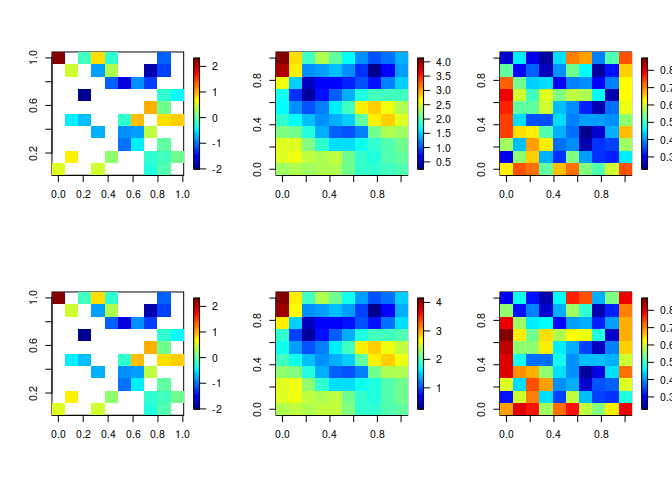### More details on likelihood evaluation

Let’s take a closer look at how the likelihood is evaluated using Vecchia. Most importantly, we can specify a parameter, $$m$$. Its value determines the number of “neighbours” of each point, or, in other words, how many other points a given point conditions on. The larger this parameter, the more accurate and expensive the approximation will be.

m=20
vecchia.approx=vecchia_specify(locs,m)
vecchia_likelihood(z,vecchia.approx,covparms,nuggets)
#>  -51.40362

Note that the function vecchia_specify determines the general properties of the approximation, but it does not depend on the data or the specific parameter values. Hence, it does not have to be re-run when searching over different parameter values in an estimation procedure.

We can also compare the results to the exact likelihood:

library(mvtnorm)
dmvnorm(z,mean=rep(0,n),sigma=Om0,log=TRUE)
#>  -51.441

In this case the approximation is very good. In general, $$m=20$$ is a good value, and $$m$$ should usually be between 10 and 40. For one-dimensional space, we can get good approximations even with $$m=5$$ or smaller.

### More details on spatial prediction

Similar to the previous section we next specify the approximation and indicate at which locations prediction is desired.

m=30
vecchia.approx=vecchia_specify(locs,m,locs.pred=locs.pred)
preds=vecchia_prediction(z,vecchia.approx,covparms,nuggets)
# returns a list with elements mu.pred,mu.obs,var.pred,var.obs,V.ord

It is also possible to print the entire predictive covariance matrix. We do it here only for the purpose of illustration. If $$n.p$$ is very large, this matrix might use up a lot of memory and we generally do not recommend plotting it directly.

Sigma=V2covmat(preds)$Sigma.pred cov.range=quantile(rbind(Sigma,cov.exact.pred),c(.01,.99)) defpar = par(mfrow=c(1,2)) fields::image.plot(cov.exact.pred,zlim=cov.range) fields::image.plot(Sigma,zlim=cov.range)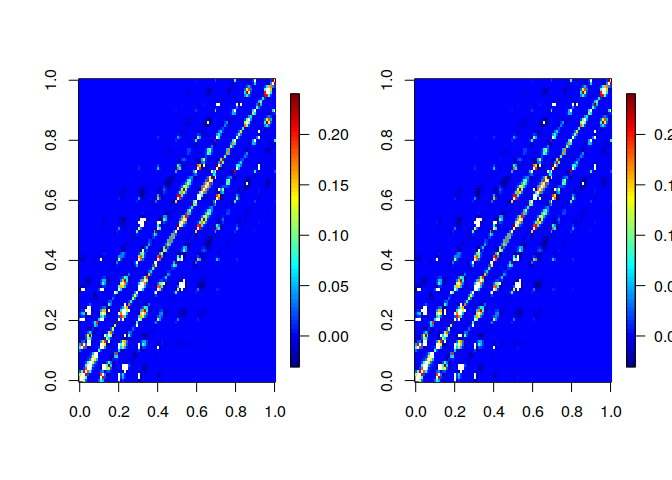par(mfrow=c(defpar)) #### Linear combinations We might sometimes be interested in a linear combination of the predicted values. In particular, we can limit our attention to only a subset of our predictions. This can be accomplished by specifying the linear combination coefficients as a matrix. As an example, we assume we are only interested in predictions at the unobserved prediction locations (not at the first n observed locations): H=Matrix::sparseMatrix(i=1:(n+n.p),j=1:(n+n.p),x=1)[(n+1):(n+n.p),] # compute variances of Hy lincomb.vars=vecchia_lincomb(H,preds$U.obj,preds$V.ord) plot(preds$var.pred,lincomb.vars)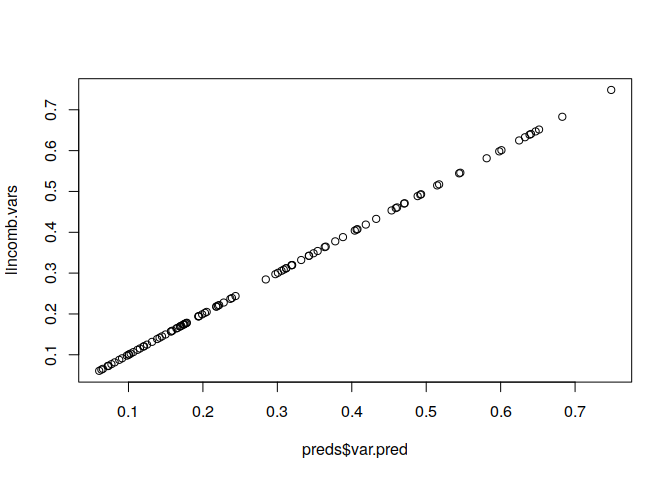As another example, we consider the overall mean of the process at all prediction locations. Using the vecchia_lincomb() function enables us to get the variance estimates easily.

mean(preds$mu.pred) #>  -0.1875505 # compute entire covariance matrix of Hy (here, 1x1) H=Matrix::sparseMatrix(i=rep(1,n.p),j=n+(1:n.p),x=1/n.p) lincomb.cov=vecchia_lincomb(H,preds$U.obj,preds$V.ord,cov.mat=TRUE) ### Other GP approximations as special cases By specifying appropriate options in vecchia_specify, we can do everything described above for several other GP approximations: Modified predictive process, FSA, MRA, latent, standard Vecchia Setting $$M=1$$ results in block full-scale approximation, specifically one with $$r_0 = \frac{m}{2}$$ knots spread over the entire domain and the remaining locations being partitioned into blocks of size $$<\frac{m}{2}+1$$. m=20 mra.options.fulls=list(M=1) blockFS = vecchia_specify(locs, m, 'maxmin', conditioning='mra', mra.options=mra.options.fulls, verbose=TRUE) #> MRA params: m=19; J=4; r=10,10; M=1 Another popular existing approximation method, modified predictive process (MPP), can also be obtained by specifying appropriate parameter settings: mra.options.mpproc=list(r=c(m,1)) MPP = vecchia_specify(locs, m, 'maxmin', conditioning='mra', mra.options=mra.options.mpproc, verbose=TRUE) #> MRA params: m=20; J=32; r=20; M=0 As we can see, MPP can be viewed as a special case of the multi-resolution approximation (MRA). A general MRA is obtained my specifying all of its three parameters mra.options.mra = list(r=c(10, 5, 5), M=2, J=2) MRA_rJM = vecchia_specify(locs, m, 'maxmin', conditioning='mra', mra.options=mra.options.mra, verbose=TRUE) #> Warning in get.mra.params(n, mra.options, m): M, r set for MRA. If #> parameter m was given, it will be overridden #> border size=1 #> border size=1 #> MRA params: m=22; J=2,2; r=10,5,7; M=2 We should note two things to note about this full specifiction of an MRA. First, providing all three $$r$$,$$J$$ and $$M$$ overrides whatever value of $$m$$ was provided. Second, in order to be able to place a knot at each point of the grid, the provided parameters might need to be adjusted. Finally, we can also use the GPvecchia package to specify a Nearest Neighbour Gaussian Process (NNGP) approximation. This can be accomplished as shown below. NNGP = vecchia_specify(locs, m, cond.yz='y') We can now easily compare different approximation methods and compare it with SGV and exact likelihood. vecchia_likelihood(z,blockFS,covparms,nuggets) #>  -51.62637 vecchia_likelihood(z,MPP,covparms,nuggets) #>  -55.6042 vecchia_likelihood(z,MRA_rJM,covparms,nuggets) #>  -51.30935 vecchia_likelihood(z,NNGP,covparms,nuggets) #>  -51.41268 vecchia_likelihood(z, vecchia_specify(locs, m), covparms, nuggets) #>  -51.40362 dmvnorm(z,mean=rep(0,n),sigma=Om0,log=TRUE) #>  -51.441 ### Non-Gaussian data Here we demonstrate how GPVecchia can fit a latent model to non-Gaussian data using the Vecchia-Laplace method. We simulate data by first generating a correlated latent field without noise, assuming the same covariance and locations generated earlier: # simulate latent process y=as.numeric(t(chol(Om0))%*%rnorm(n)) Then we sample a single non-Gaussian value for each latent value. The variability introduced by the sampling induces heteroskedasticity, in contrast the the constant noise added to the Gaussian case. Below we use a logistic model for binary data, but there are implementations for count and continuous positive (right-skewed) data as well. data.model = "logistic" # simulate data if(data.model=='poisson'){ z = rpois(n, exp(y)) } else if(data.model=='logistic'){ z = rbinom(n,1,prob = exp(y)/(1+exp(y))) } else if(data.model=='gamma'){ z = rgamma(n, shape = default_lh_params$alpha, rate = default_lh_params$alpha*exp(-y)) }else{ print('Error: Distribution not implemented yet.') } # plot simulated data, 1 or 2D defpar = par(mfrow=c(1,2)) if(spatial.dim==1) { plot(locs,y, main = "latent") plot(locs,z, main = "observed") } else { fields::quilt.plot(locs,y, main = "Latent") fields::quilt.plot(locs,z, main = "Observed") }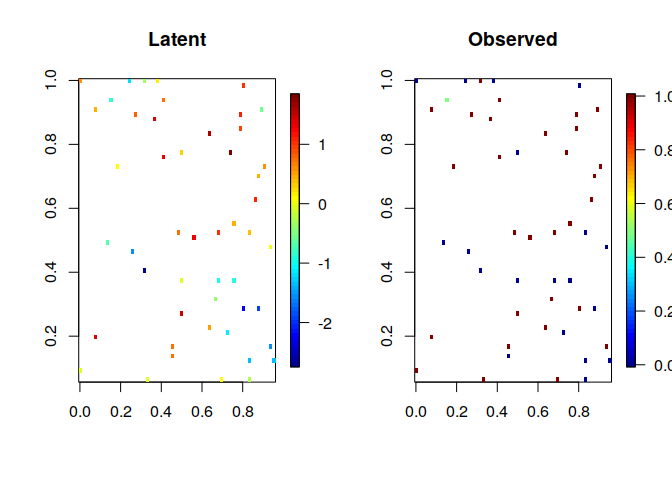par(defpar) Given the simulated data, we now can efficiently estimate the latent field by specifying the number of conditioning points $$m$$ described earlier. Interweaved ordering is best for 1D data while response-first (‘zy’) ordering is best for higher dimensions. m=10 if(spatial.dim==1){ vecchia.approx=vecchia_specify(locs,m) #IW ordering } else { vecchia.approx=vecchia_specify(locs,m,cond.yz='zy') #RF ordering } With the approximated covariance structure, we can calculate the posterior estimate for the latent field using the Vecchia-Laplace method and plot the result. Pure Laplace approximation is included for comparison; even with a small value for $$m$$, we can get a result similar to Laplace but with much lower cost. posterior = calculate_posterior_VL(z,vecchia.approx,likelihood_model=data.model, covparms = covparms) if (spatial.dim==1){ par(mfrow=c(1,1)) ord = order(locs) # order so that lines appear correctly y_limits = c(min(y, posterior$mean[ord]), max(y, posterior$mean[ord])) plot(locs[ord], y[ord], type = "l", ylim = y_limits ) lines(locs[ord], posterior$mean[ord], type = "l", col=3, lwd=3)
legend("bottomright", legend = c("Latent", "VL"), col= c(1,3), lwd=c(1,3))
} else if (spatial.dim==2){
dfpar = par(mfrow=c(1,2))
# ordering unnecessary; we are using a scatter plot rather than lines
quilt.plot(locs, y, main= "Truth")
quilt.plot(locs, posterior$mean, main= "VL m=10") par(defpar) }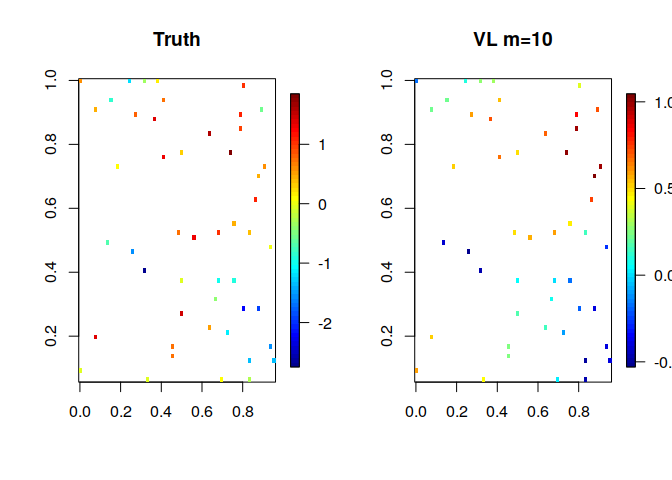### Non-Gaussian predictions Predictions are computed as before, using Vecchia-Laplace methods where needed ###### specify prediction locations ####### n.p=30^2 if(spatial.dim==1){ # 1-D case locs.pred=matrix(seq(0,1,length=n.p),ncol=1) } else { # 2-D case grid.oneside=seq(0,1,length=round(sqrt(n.p))) locs.pred=as.matrix(expand.grid(grid.oneside,grid.oneside)) # grid of pred.locs } n.p=nrow(locs.pred) ###### specify Vecchia approximation ####### vecchia.approx.pred = vecchia_specify(locs, m=10, locs.pred=locs.pred) ### carry out prediction preds = vecchia_laplace_prediction(posterior, vecchia.approx.pred, covparms) # plotting predicitions if (spatial.dim==1){ defpar = par(mfrow=c(1,1)) ord = order(locs) # order so that lines appear correctly plot(locs[ord], y[ord], type = "l", xlim=c(0,1.2), ylim = c(-1,3)) lines(locs, posterior$mean, type = "p", col=4, lwd=3, lty=1)
lines(locs.pred, preds$mu.pred, type = "l", col=3, lwd=3, lty=1) lines(locs.pred,preds$mu.pred+sqrt(preds$var.pred), type = "l", lty = 3, col=3) lines(locs.pred,preds$mu.pred-sqrt(preds$var.pred), type = "l", lty = 3, col=3) legend("topleft", legend = c("Latent", "VL: Pred", "VL: 1 stdev"), col= c(1,3,3), lwd=c(1,2,1), lty = c(1,1,3)) par(defpar) } else if (spatial.dim==2){ defpar = par(mfrow=c(1,2)) # ordering unnecessary; we are using a scatter plot rather than lines quilt.plot(locs, y, main= "True Latent", xlim = c(0,1), ylim = c(0,1), nx=64, ny=64) quilt.plot(locs.pred, preds$mu.pred,  main= "VL Prediction",nx = 30, ny=30)
par(defpar)
}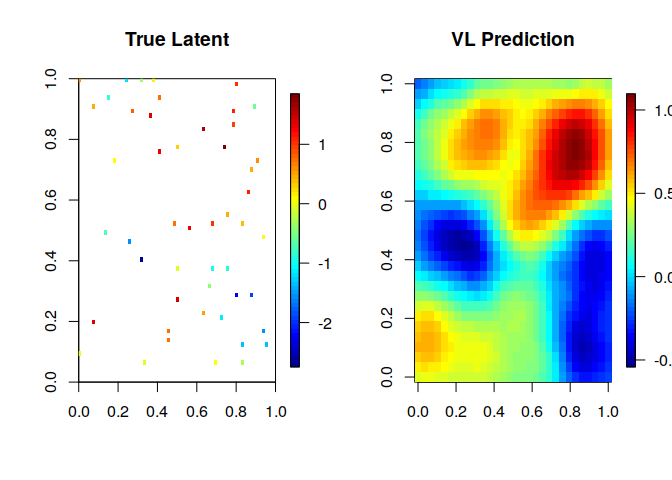### Parameter estimation

The likelihood of the data for a set of parameters can be computed efficiently using the command below.

vecchia_laplace_likelihood(z,vecchia.approx,likelihood_model=data.model,covparms = covparms)
#>  -34.876

This can be used for parameter estimation by evaluating the likelihood over a grid of parameter values or in an iterative optimization method such as Nelder-Mead. Reparameterizing the parameters improves performance.

# currently set up for covariance estimation
vecchia.approx=vecchia_specify(locs, m=10, cond.yz = "zy") # for posterior
vecchia.approx.IW = vecchia_specify(locs, m=10) # for integrated likelihood
if (spatial.dim==1) vecchia.approx=vecchia.approx.IW

vl_likelihood = function(x0){
theta = exp(x0)
covparms=c(theta, theta, theta) # sigma range smoothness
prior_mean = 0 # can be a parameter as well
# Perform inference on latent mean with Vecchia Laplace approximation
vll = vecchia_laplace_likelihood(z,vecchia.approx, likelihood_model=data.model,
covparms, return_all = FALSE,
likparms = default_lh_params, prior_mean = prior_mean,
vecchia.approx.IW = vecchia.approx.IW)
return(-vll)

}
x0 = log(c(.07,1.88, 1.9))
vl_likelihood(x0)
# Issues with R aborting, maxit set to 1
res = optim(x0, vl_likelihood, method = "Nelder-Mead", control = list("trace" = 1, "maxit" = 1))
exp(res\$par[1:3])
vl_likelihood(x0)